# Evaluation of the Results

## Cross-Comparison of Experiments

The 2D PIV results were checked with 1D LDA measurements. A satisfactory agreement was found as can be seen in the following figures (Re=10,600) [Rapp et al. (2009), Rapp (2009)]. The LDA measurement accuracy depends on the frequency, the number of samples and the window functions. It can be quantified to <0.01 u. The assessment of the measurement accuracy for PIV measurements is rather complicated. The laser sheet and camera adjustment, the subpixel interpolation and the validation algorithm have an impact on the measurement. In general the accuracy for PIV measurements is about 0.05 pixel which leads to 0.00625 ub. In this case two independently operating measurement techniques were compared with each other and only minor deviations were identified (see Fig. 4.1).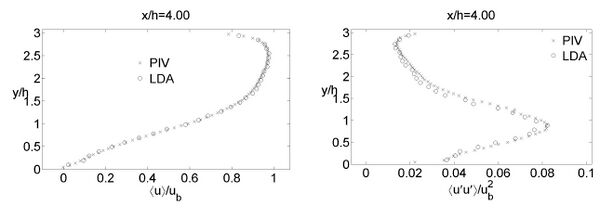Fig. 4.1 Mean streamwise velocity component $\langle u\rangle /u_{b}$(left) and mean normal stress $\langle u\prime u\prime \rangle /u_{b}^{2}$The PIV field data and the point by point LDA measurements show an excellent accordance. The mean velocity component in the x-direction and the streamwise Reynolds stress show only minor deviations that can be traced back to the measurement procedure. As the LDA data could only be acquired by measuring the particular points periodically and at different instants of time, the profiles obtained by LDA turn out to be less smooth than the ones obtained by PIV.

The periodicity and the homogeneity of the flow could be shown for Re≥10,600 [Rapp et al. (2009), Rapp (2009)]. The following plots (Fig. 4.2) show the mean streamwise and vertical velocity component at x/h=4.0 between hills six and seven and between hills seven and eight for Re=10,600. Only minor deviations between the two segments can be detected. Within the whole flow field the greatest difference of the mean streamwise velocity component between the two consecutive segments was found at x/h=4.0, y/h=1.1 and can be quantified to be $\Delta u=0.012u_{b}$. The corresponding location and magnitude for the vertical component are: x/h=3.0, y/h=0.55, $\Delta v=0.008u_{b}$.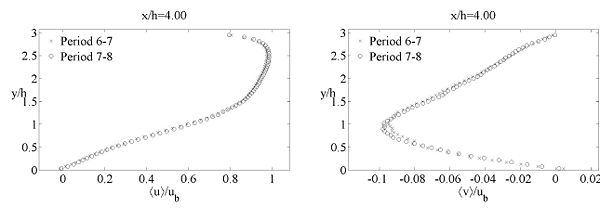Fig. 4.2 Mean streamwise velocity component $\langle u\rangle /u_{b}$(left) and mean vertical velocity component $\langle v\rangle /u_{b}$The mean lateral component w was found to be smaller than |0.01| ub within $-5\leq z/h\leq 0$on the left channel half for Re≥10600. The greatest variations of the rms values remain below 0.005 ub from Re≥10600 on. The contribution of these lateral variations on the momentum balance were investigated in Rapp (2009). $\partial \langle u\prime w\prime \rangle /\partial z$has the greatest impact on the momentum balance in the x-direction in the center plane at y/h=1.53 (Re=10,600); it oscillates around zero with magnitudes between $-1,66\cdot 10^{-3}u_{b}^{2}/h$and $1,64\cdot 10^{-3}u_{b}^{2}/h$. Insufficient statistical averaging was suspected to be the reason for these oscillations in the velocity/rms gradients. However, $\partial \langle u\prime v\prime \rangle /\partial y$is one order of magnitude greater but still smaller than the dominating terms $u\partial \langle u\rangle /\partial x$and $v\partial \langle u\rangle /\partial y$. Further investigations on the periodicity of the flow in the experiment as well as a detailed analysis of the homogeneity can be found in Rapp et al. (2009) and Rapp (2009).

## Cross-Comparison of CFD Calculations

A cross-comparison of the results achieved by the different methods was carried out. For that purpose two different Reynolds numbers were considered. According to Table 1 two DNS predictions are available at Re = 2800. Case 4 was performed by LESOCC using a curvilinear grid and case 5 is based on MGLET using a Cartesian grid with about 3.5 times more grid points. At Re = 5600 case 7 is taken into account which denotes a wall-resolved LES prediction on the curvilinear grid. These results are compared with case 8 providing numerical data on an extremely fine Cartesian grid (231 million grid points) which is beyond all doubt a well-resolved DNS. The cross-comparison was also conducted for Re = 1400. However, since no new findings compared to the outcome presented in the following sections resulted, for the sake of brevity this Reynolds number is omitted here.

Figures 4.3 to 4.6 show exemplarily the distribution of the averaged streamwise velocity $\langle U\rangle /U_{B}$at four different vertical positions in the flow field, i.e. x / h = 0.5, 2, 4, and 6. The first position is located shortly after the separation line and crosses the strong shear layer; the second profile is at the beginning of the flat floor and hence within the main recirculation region. The third one is located near the end of the recirculation bubble and finally the last is positioned behind the main separation region in the reattached flow. At both Re the agreement observed between the results obtained by the different numerical methods is excellent. Only marginal deviations are found. For example, MGLET predicts slightly larger back-flow velocities at x / h = 4 for Re = 2800 compared with the data of LESOCC. This marginal deviation is still visible further downstream at x / h = 6 close to the lower wall. Besides that the profiles resulting from the two independent simulation techniques lie on top of each other at both Re.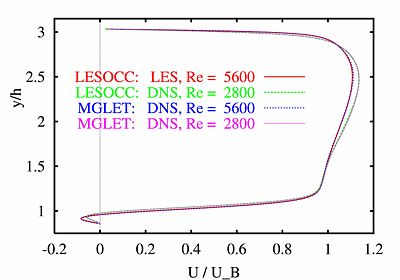Fig. 4.3 Comparison of predictions by LESOCC and MGLET based on the streamwise velocity profiles at x / h = 0.5 and two different Reynolds numbers, i.e. Re = 2800 (case 4 and 5) and 5600 (case 7 and 8, see Table 1)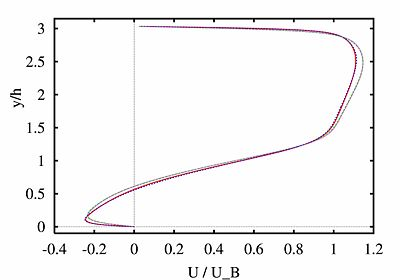Fig. 4.4 Comparison of predictions by LESOCC and MGLET based on the streamwise velocity profiles at x / h = 2. and two different Reynolds numbers, i.e. Re = 2800 (case 4 and 5) and 5600 (case 7 and 8, see Table 1)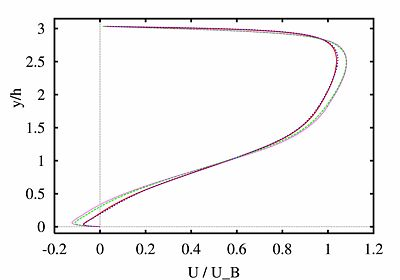Fig. 4.5 Comparison of predictions by LESOCC and MGLET based on the streamwise velocity profiles at x / h = 4. and two different Reynolds numbers, i.e. Re = 2800 (case 4 and 5) and 5600 (case 7 and 8, see Table 1)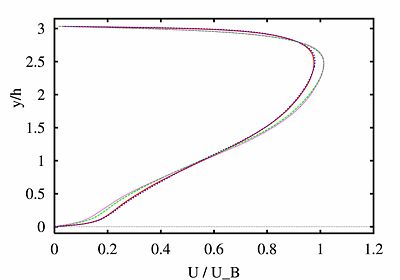Fig. 4.6 Comparison of predictions by LESOCC and MGLET based on the streamwise velocity profiles at x / h = 6. and two different Reynolds numbers, i.e. Re = 2800 (case 4 and 5) and 5600 (case 7 and 8, see Table 1)

Furthermore, Figs. 4.7 to 4.12 display the resolved Reynolds stresses ${\langle u'u'\rangle /U_{B}^{2}}$, ${\langle v'v'\rangle /U_{B}^{2}}$, and ${\langle u'v'\rangle /U_{B}^{2}}$at two locations. The two main observations are as follows. First, a clear trend is obvious concerning the variation of the Reynolds stresses with varying Reynolds numbers. Second, a reasonable agreement is found between the predictions based on the two different codes at both Re. Similar to the normal velocity component $\langle V\rangle$small deviations are visible near the extrema occurring in the free shear layer. Except in this narrow region the normal stresses and the shear stress belonging to a certain Re agree satisfactorily. The extremely well-resolved prediction by MGLET at Re = 5600 (case 8 in Table 1) shows the largest peak values for ${\langle u'u'\rangle /U_{B}^{2}}$. The extrema of the normal stress ${\langle v'v'\rangle /U_{B}^{2}}$as well as the shear stress ${\langle u'v'\rangle /U_{B}^{2}}$in the shear layer (x/h = 2) are also slightly larger than the results predicted by LESOCC (case 7). The deviations found at this Reynolds number can be explained by the fact that the last is an LES prediction whereas MGLET provides DNS data. The modeled stress contributions, although known to be small, are not taken into account in the LES data at Re = 5600 in Figs. 4.7 to 4.12.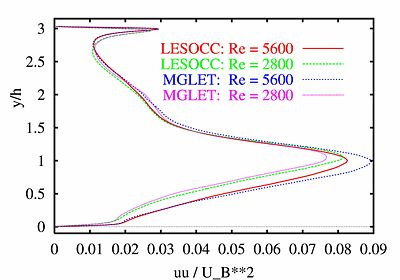Fig. 4.7 Comparison of predictions by LESOCC and MGLET based on the streamwise normal Reynolds stress at x/h = 2.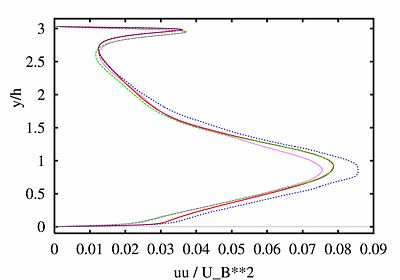Fig. 4.8 Comparison of predictions by LESOCC and MGLET based on the streamwise normal Reynolds stress at x/h = 4.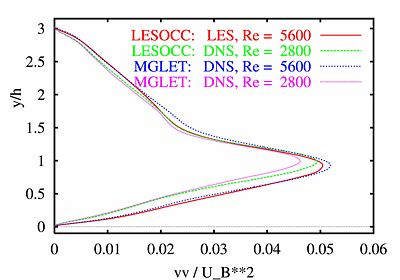Fig. 4.9 Comparison of predictions by LESOCC and MGLET based on the wall-normal Reynolds stress at x/h = 2.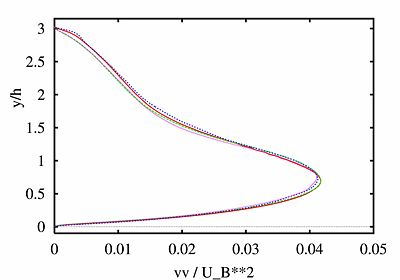Fig. 4.10 Comparison of predictions by LESOCC and MGLET based on the wall-normal Reynolds stress at x/h = 4.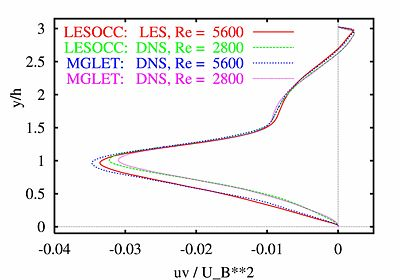Fig. 4.11 Comparison of predictions by LESOCC and MGLET based on the Reynolds shear stress at x/h = 2.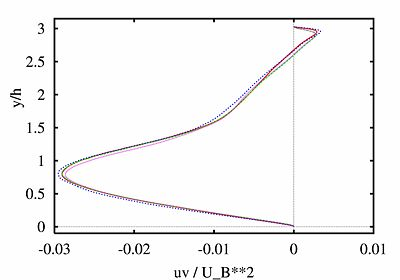Fig. 4.12 Comparison of predictions by LESOCC and MGLET based on the Reynolds shear stress at x/h = 4.

More comparisons including wall shear stresses and pressure distributions can be found in Breuer et al. (2009).

## Cross-Comparison of CFD Calculations with Literature Data at Re = 10,595

In this section, for Re= 10,595, the wall-resolved LES prediction by LESOCC (case 9) is compared with the literature data by Fröhlich et al. (2005). For this comparison one should bear in mind that both simulations are based on similar numerical techniques (second-order accurate in space and time using curvilinear grids), but the main issues from the section about the resolution should be recapitulated. The present grid consists of about three times more grid points than that used in Fröhlich et al. (2005) whereby the increased number of points is especially placed in the wall-normal direction to resolve also the upper wall and thus to avoid wall functions.

Figures 4.13 to 4.16 depict a comparison at three different locations (x/h = 0.5, 2, and 6) showing averaged velocity profiles for $\langle U\rangle /U_{B}$and $\langle V\rangle /U_{B}$as well as turbulent quantities such as the resolved turbulent kinetic energy $\langle k\rangle /U_{B}^{2}=0.5(\langle u'u'\rangle +\langle v'v'\rangle +\langle w'w'\rangle )/U_{B}^{2}$and the shear stress $\langle u'v'\rangle /U_{B}^{2}$. For the streamwise velocity component $\langle U\rangle /U_{B}$it is obvious that a very good agreement can be observed in the lower part of the computational domain close to the lower wall. Solely in the vicinity of the upper wall deviations are visible which can be assigned to the application of wall functions in Fröhlich et al. (2005). The streamwise velocity component $\langle U\rangle /U_{B}$is underpredicted here and shows a non-smooth behavior. The resolution was quite coarse in that case in Fröhlich et al. (2005) partially leading to wiggles in the solution as is obvious in Fig. 4.14 for the wall-normal velocity component $\langle V\rangle /U_{B}$. The deficits resulting from the usage of wall functions at the upper wall are also detectable in the turbulent quantities, e.g. the location of the peak value of $\langle k\rangle /U_{B}^{2}$is shifted away from the upper wall and the shear stress profile $\langle u'v'\rangle /U_{B}^{2}$strongly deviates from the wall-resolved LES prediction (case 9) in this region. Since the total mass flux through the channel was fixed, the underprediction of $\langle U\rangle /U_{B}$in the vicinity of the upper wall has a certain influence on the overall flow development. Nevertheless, the agreement between the present wall-resolved LES prediction (case 9) and the literature data (Fröhlich et al. 2005) is satisfactory and further validates the former.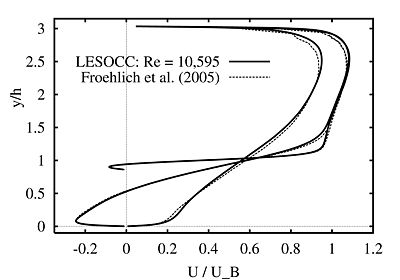Fig. 4.13 Comparison of LES predictions by LESOCC at Re = 10,595 (case 9 in Table 1) and literature data by Fröhlich et al. (2005) at three different locations (x / h = 0.5, 2, and 6); streamwise velocity.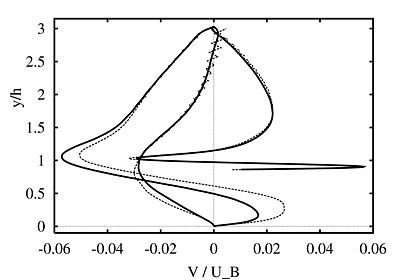Fig. 4.14 Comparison of LES predictions by LESOCC at Re = 10,595 (case 9 in Table 1) and literature data by Fröhlich et al. (2005) at three different locations (x / h = 0.5, 2, and 6); normal velocity.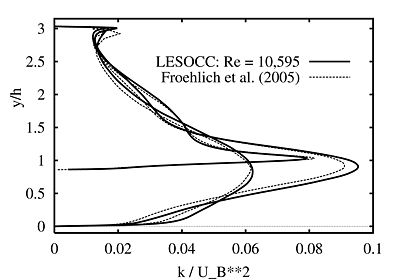Fig. 4.15 Comparison of LES predictions by LESOCC at Re = 10,595 (case 9 in Table 1) and literature data by Fröhlich et al. (2005) at three different locations (x / h = 0.5, 2, and 6); resolved turbulent kinetic energy $\langle k\rangle /U_{B}^{2}$.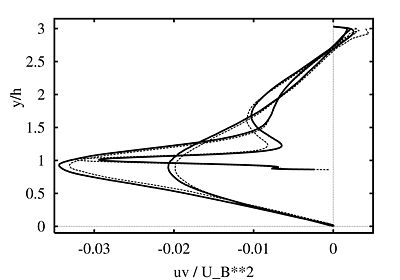Fig. 4.16 Comparison of LES predictions by LESOCC at Re = 10,595 (case 9 in Table 1) and literature data by Fröhlich et al. (2005) at three different locations (x / h = 0.5, 2, and 6); resolved shear stress $\langle {u'v'}\rangle /U_{B}^{2}$.

## Comparison of LES with Experiments at Re = 10,595

The experimental data at Reynolds number 10,595 are compared with the highly resolved LES results by LESOCC [Breuer et al. (2009), Rapp et al. (2009)]. The following figures show averaged velocity profiles for <u>/uB at four streamwise positions.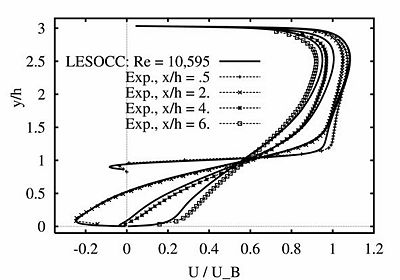Fig. 4.17 Mean streamwise velocity component $/U_{B}$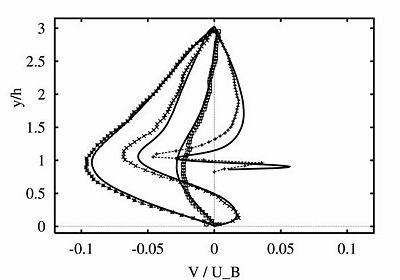Fig. 4.18 Mean streamwise velocity component $/U_{B}$The agreement between the predicted and the measured mean streamwise velocity component in the x-direction is very good. Minor deviations can be found in the shear layer (slightly higher velocities in the experiment) and in the post-reattachment zone. The vertical velocity component <v>/ub is about one order of magnitude smaller than the streamwise component and is more sensitive. However, the agreement between calculations and measurements is also fully satisfactory for this componenet. The largest deviations are found at x/h=2.

The measured and the predicted Reynolds stresses in Figs. 4.19 - 4.21 show a close agreement at the different x/h-positions. The location of the experimental peak values and their distributions are in close accordance with the predictions. Solely at x/h=6 slightly higher streamwise normal and shear stresses were found in the experimental data. Nevertheless, the agreement between the PIV measurement and the LES prediction is highly satisfactory.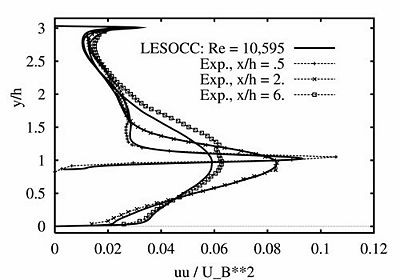Fig. 4.19 Mean Reynolds normal stress $/U_{B}^{2}$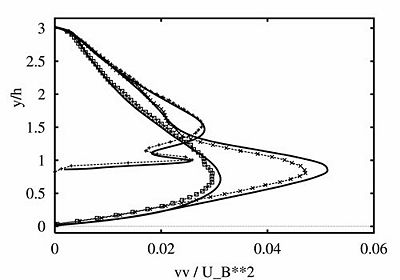Fig. 4.20 Mean Reynolds normal stress $/U_{B}^{2}$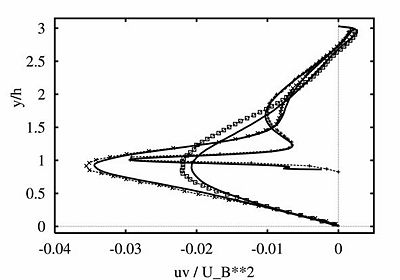Fig. 4.21 Mean Reynolds shear stress $/U_{B}^{2}$At Re = 5600 the DNS data achieved by MGLET (case 8 in Table 1) were compared to the PIV measurements. For the mean streamwise and wall-normal velocity a similar agreement between the predictions and the measurements as observed at Re = 10,595 was found. The situation is slightly different for the Reynolds stresses. During the measurements it was found that the determination of the stresses is becomes more and more challenging with decreasing Re. For the case of Re = 5600 this results in the profiles of the Reynolds stresses to be similarly shaped, but the experimentally determined stresses exceed the numerical ones (case 8 in Table 1) systematically by up to about 20%. Initially, pump fluctuations were considered to be the reason for that, but high-pass filtering excluded this cause. Up to now, the reason for the deviations in the Reynolds stresses at Re = 5600 could not be found.

In conclusion, the various cross-comparisons carried out have demonstrated that the predicted data are reliable.

## Separation and Reattachment Lengths

The flow separates shortly behind the hill crest at all Re. As shown in Fig.~4.22 the separation point moves upstream from $x_{S}/h\approx 0.45$at Re = 100 to $x_{S}/h\approx 0.3$at Re = 700 and finally to $x_{S}/h\approx 0.18$at Re = 5600 but then settles down at a slightly larger value of $x_{S}/h\approx 0.19$at Re = 10,595. The undulations in the wall shear stress at the beginning of the main recirculation bubble seem to be geometry related and not much affected by Reynolds number effects. This statement is supported by the observation that the undulations are visible in all simulations using LESOCC but also for the predictions based on MGLET.

Past the hill the main recirculation region follows with a nearly constant pressure plateau in a large part of the separation bubble. The reattachment position $x_{R}/h$displayed decreases from Re = 100 to 1400. Between Re = 1400 and Re = 2800 a sudden change can be observed in Fig.~4.9. The reattachment length $x_{R}/h$increases again in this narrow Re-range. Afterwards, a clear trend concerning the reattachment length is visible for $Re\geq 2800$where $x_{R}/h$decreases again with increasing Re. Since this phenomenon is consistently predicted by both independent codes, it is assumed to be no numerical artifact. The corresponding values of the reattachment lengths are ${x_{R}/h=5.24,5.19,5.41,5.09}$, and ${4.69}$for Re = 700 to 10,595, respectively. A similar behavior of the reattachment length with increasing Re has been observed for the backward-facing step flow by Armaly et al. (1983). This observation also explains why with increasing Re there is no continuous trend visible for the averaged pressure distribution.

Interestingly, a small region with positive averaged wall shear stress is found within the main separation bubble at Re = 1400 by both codes (case 2 and 3 in Table 1). That leads to a tiny counterclockwise rotating structure in the region x/h = 0.6 to 0.8 at the falling edge of the hill not depicted here. Contrarily, at the higher Re numbers the wall shear stress is negative in the entire recirculation region and thus no such tiny structure is detected. This special feature solely observed at Re = 1400 might be an explanation for the non-monotonous behavior of the reattachment length x_R / h. However, the interpretation has to be done with care, since one has to bear in mind that the data presented are averaged in time and in homogeneous direction. Animations of the flow field at the low Reynolds number cases have clearly shown that along the falling edge of the hill intermittently attached flow regions can be detected which explain the positive wall shear stress.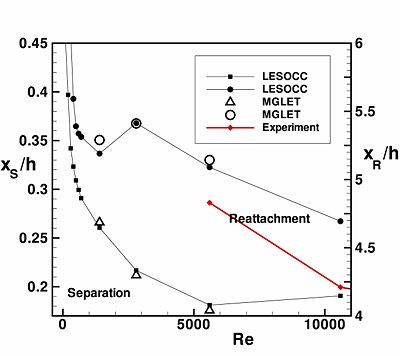Fig. 4.22 Separation and reattachment lengths versus Reynolds number; comparison of predictions by LESOCC and MGLET $(100\leq Re\leq 10,595)$In the experiment only the reattachment lengths are determined.

Fig. 4.23 despicts the separation and reattachment lengths for a wider Reynolds number range and with another scaling of the axes. In the experiment it was not possible to determine the separation point reliably. Thus only the reattachment length is shown. The general trend of a decreasing reattachment length with increasing Re found in the simulations is confirmed by the experimental data.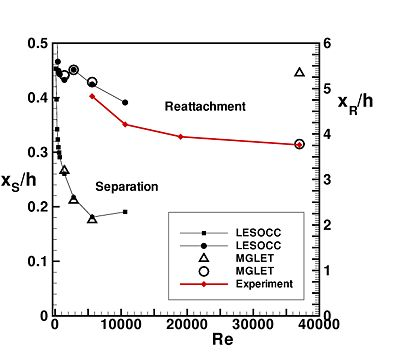Fig. 4.23 Separation and reattachment lengths versus Reynolds number; comparison of predictions by LESOCC and MGLET $(100\leq Re\leq 37,000)$In the experiment only the reattachment lengths are determined.

# Flow Development with increasing Reynolds number

Figure 4.24 shows a visualization of the instantaneous flow field via the vorticity component $\omega _{z}$normal to the cross-section for a series of prediction between Re = 100 and 10,595. At the lowest Reynolds number considered, the flow is found to reach steady-state after about 100 dimensionless time units which is equivalent to about 11 flow-through times. The flow separates past the hill crest at about x/h = 0.45 and forms a large recirculation region which fills the lower portion of the entire constriction completely. Thus reattachment is observed in this case at the rising edge of the second hill at about x/h = 7.73. No three-dimensional structures can be detected. The situation changes completely when the Reynolds number is increased to Re = 200. Now and for all other higher Re the flow remains unsteady all the time. Up to about 140 dimensionless time units the flow field at Re = 200 is two-dimensional. However, after this initial phase first three-dimensional flow structures develop which are detected by monitoring the spanwise velocity component W. With increasing Re the initial two-dimensional stage is shortened so that at Re = 700 first three-dimensional phenomena can be found at about 50 dimensionless time units past the initial stage. As visible with increasing Re the spatial structures observed become smaller and smaller.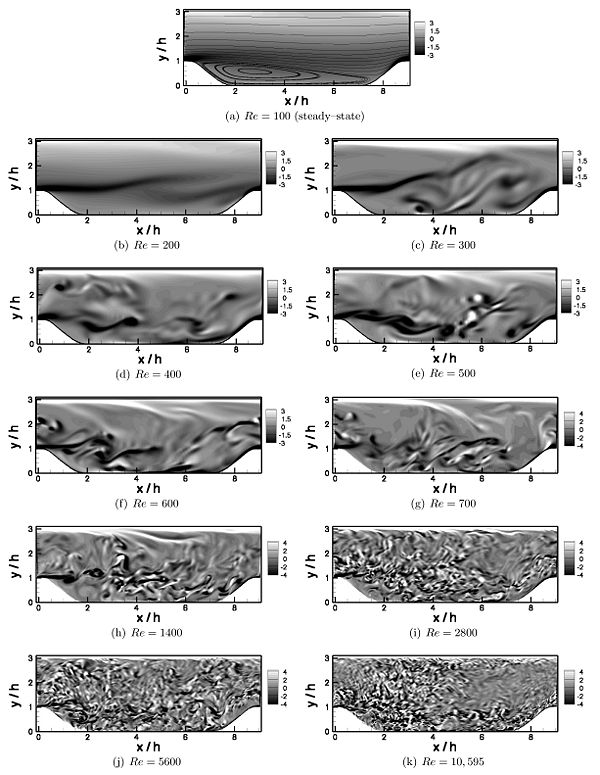Fig. 4.24 Comparison of (instantaneous) vorticity component $\omega _{z}$normal to the cross-section at eleven different Reynolds numbers (Re = 100 to 10,595) at an arbitrarily chosen instant in time

# Results from ATAAC Project

In D3.2-36_Jakirlic-ST01-ERCOFTAC-WIKI.pdf results obtained in the ATAAC project with various RANS and Hybrid LES-RANS methods are presented in graphical form. For the Reynolds number 10595 they are compared with the LESOCC results presented above (case 9 in Table 1) and for Re=37,000 among themselves.

Contributed by: (*) Christoph Rapp, (**) Michael Breuer, (*) Michael Manhart, (*) Nikolaus Peller — (*) Technische Universität München, (**) Helmut-Schmidt Universität Hamburg